Home Practice
For learners and parents For teachers and schools
Textbooks
Full catalogue
Pricing SupportLog in

We think you are located in United States. Is this correct?

# 12.2 The photoelectric effect

## 12.2 The photoelectric effect (ESCQJ)

Around the turn of the twentieth century, it was observed by a number of physicists (including Hertz, Thomson and Von Lenard) that when light was shone onto a metal plate, electrons were emitted by the metal. This is called the photoelectric effect. (photo for light, electric for the electron.)

The characteristics of the photoelectric effect were a surprise and a very important development in modern Physics. To understand why it was a surprise we need to look at the history to understand what physicists were expecting to happen and then understand the implications for Physics going forward.

### History and expectations as to the photoelectric effect (ESCQK)

In 1887, Heinrich Hertz (a German physicist) noticed that ultraviolet light incident on a metal plate could cause sparks. Metals were known to be good conductors of electricity, because the electrons are more able to move. They should be able to be dislodged if energy were added through the incident light. The problem was that different metals requred different minimum frequencies of light.

The expectation at the time was that electrons would be emitted for any frequency of light, after a delay (for low intensities) during which the electrons absorbed sufficient energy to escape from the metal surface. The higher the intensity the shorter the delay was be as they would absorb energy faster. This was based on the idea that light was a wave continuously delivering energy to the electrons.

It is important to remember that higher frequency light corresponds to higher energy.

The next piece of the puzzle came from Philipp Lenard (a Hungarian physicist) in 1902 when he discovered that the maximum velocity with which electrons are ejected by ultraviolet light is entirely independent of the intensity of light.

His expectation was that at high intensities the electrons would absorb more energy and so would have a greater velocity.

A paradox existed as the expectations and the observations did not match.

Albert Einstein (a German physicist) solved this paradox by proposing that light is made up of packets of energy called quanta (now called photons) which interacted with the electrons in the metal like particles instead of waves. Each incident photon would transfer all its energy to one electron in the metal.

The photoelectric effect

The photoelectric effect is the process whereby an electron is emitted by a substance when light shines on it.

Einstein received the 1921 Nobel Prize for his contribution to understanding the photoelectric effect. His explanation wasn't very popular and took a while to be accepted, in fact, some scientists at the time felt that is was a big mistake.

In the motivation letter for Einstein to be accepted into the Prussian Academy of Science it was specifically mentioned as a mistake:

In sum, one can say that there is hardly one among the great problems in which modern physics is so rich to which Einstein has not made a remarkable contribution. That he may sometimes have missed the targeting his speculations, as, for example, in his hypothesis of light-quanta, cannot really be held too much against him, for it is not possible to introduce really new ideas even in the most exact sciences without sometimes taking a risk - A. Pais, “Subtle is the Lord: The Science and the Life of Albert Einstein,” New York: Oxford University Press, 1982, p. 382

### Implications of Einstein's model (ESCQM)

For a paper on the history of the photoelectric effect and the analysis of its treatment in a variety of textbooks see: http://onlinelibrary.wiley.com/doi/10.1002/sce.20389/pdf

Einstein's model is consistent with the observation that the electrons were emitted immediately when light was shone on the metal and that the intensity of the light made no difference to the maximum kinetic energy of the emitted electrons.

The energy needed to knock an electron out of the substance is called the work function (symbol $$W_{0}$$) of the substance. This is a characteristic of the substance. If the energy of the photon is less than the work function then no electron can be emitted, no matter how many photons strike the substance. We know that the frequency of light is related to the energy, that is why there is a minimum frequency of light that can eject electrons. This minimum frequency we call the cut-off frequency, $$f_0$$. For a specific colour of light (i.e. a certain frequency or wavelength), the energy of the photons is given by $$E=hf=\frac{hc}{\lambda}$$, where $$h$$ is Planck's constant. This tells us that the $$W_{0} = hf_0$$ .

Work function
The minimum energy needed to knock an electron out of a metal is called the work function (symbol $$W_{0}$$) of the metal. As it is energy, it measured in joules (J).

Energy is conserved so if the photon has a higher energy than $$W_{0}$$ then the excess energy goes into the kinetic energy $${E}_{k}$$ of the electron that was emitted from the substance.

The excess over and above the binding energy is actually the maximum kinetic energy the emitted electron can have. This is because not all electrons are on the surface of the substance. For electrons below the surface there is additional energy required to eject the electron from the material which then cannot contribute to the kinetic energy of the electron.

\begin{align*} E & = W_{0}+{E}_{k\max} \\ {E}_{k\max}& = hf-W_{0} \end{align*}

This equation is known as the photoelectric equation.

The last piece of the puzzle is now clear, the question was `why does increasing the intensity of the light not affect the maximum kinetic energy of the emitted photons?'. The answer is that each emitted electron has absorbed one photon, increasing the intensity just increases the number of photons (we expect more electrons but we don't expect their maximum kinetic energy to change).

The discovery and understanding of the photoelectric effect was one of the major breakthroughs in science in the twentieth century as it provided concrete evidence of the particle nature of light. It overturned previously held views that light was composed purely of a continuous transverse wave. On the one hand, the wave nature is a good description of phenomena such as diffraction and interference for light, and on the other hand, the photoelectric effect demonstrates the particle nature of light. This is now known as the 'dual-nature' of light. (dual means two)

Einstein won the 1921 Nobel Prize for Physics for this quantum theory and his explanation of the photoelectric effect.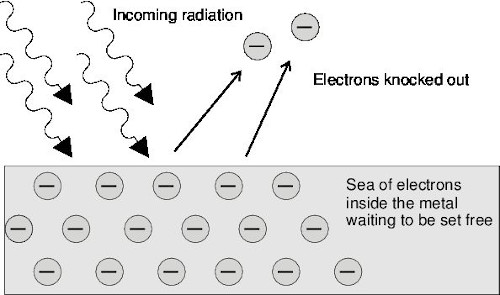Figure 12.1: The photoelectric effect: Incoming photons on the left hit the electrons inside the metal surface. The electrons absorb the energy from the photons, and are ejected from the metal surface.

We can observe this effect in the following practical demonstration of photoelectric emission. A zinc plate is charged negatively and placed onto the cap of an electroscope. In Figure 12.2, red light is shone onto the zinc plate. There is no change observed even if the intensity (brightness) of the red light is increased. In Figure 12.3, ultraviolet light of low intensity is shone onto the zinc and it is observed that the leaf of the electroscope collapse. This allows us to conclude that the negative charge on the plate decreased as electrons were ejected from the metal when the ultraviolet light was incident on the plate.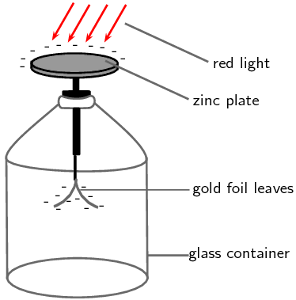Figure 12.2: Red light incident on the zinc plate of an electroscope.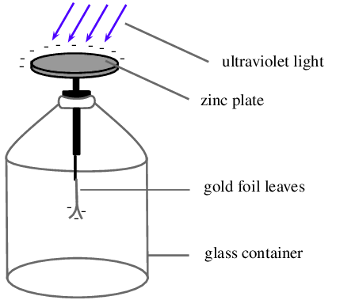Figure 12.3: Ultraviolet light incident on the zinc plate of an electroscope.

The work function is different for different elements. The smaller the work function, the easier it is for electrons to be emitted from the metal. Metals with low work functions make good conductors. This is because the electrons are attached less strongly to their surroundings and can move more easily through these materials. This reduces the resistance of the material to the flow of current i.e. it conducts well. Table 12.1 shows the work functions for a range of elements.

 Element Work Function (J) Aluminium $$\text{6,9} \times \text{10}^{-\text{19}}$$ Beryllium $$\text{8,0} \times \text{10}^{-\text{19}}$$ Calcium $$\text{4,6} \times \text{10}^{-\text{19}}$$ Copper $$\text{7,5} \times \text{10}^{-\text{19}}$$ Gold $$\text{8,2} \times \text{10}^{-\text{19}}$$ Lead $$\text{6,9} \times \text{10}^{-\text{19}}$$ Silicon $$\text{1,8} \times \text{10}^{-\text{19}}$$ Silver $$\text{6,9} \times \text{10}^{-\text{19}}$$ Sodium $$\text{3,7} \times \text{10}^{-\text{19}}$$

Table 12.1: Work functions of selected elements determined from the photoelectric effect. (From the Handbook of Chemistry and Physics.)

temp text

### Units of energy (ESCQN)

When dealing with calculations at a small scale (like at the level of electrons) it is more convenient to use different units for energy rather than the joule (J). We define a unit called the electron-volt (eV) as the kinetic energy gained by an electron passing through a potential difference of one volt.

$$E=q\times V$$

where q is the charge of the electron and V is the potential difference applied. The charge of 1 electron is $$\text{1,6} \times \text{10}^{-\text{19}}$$ $$\text{C}$$, so $$\text{1}$$ $$\text{eV}$$ is calculated to be:

$$1 \mathrm{eV}=\left(\text{1,6} \times \text{10}^{-\text{19}}\text{ C} \times \text{1}\text{ V} \right)= \text{1,6} \times \text{10}^{-\text{19}}\text{ J}$$

You can see that $$\text{1,6} \times \text{10}^{-\text{19}}$$ $$\text{J}$$ is a very small amount of energy and so using electron-volts (eV) at this level is easier.

Hence, $$\text{1}$$ $$\text{eV}$$ = $$\text{1,6} \times \text{10}^{-\text{19}}$$ $$\text{J}$$ which means that $$\text{1}$$ $$\text{J}$$ = $$\text{6,241} \times \text{10}^{\text{18}}$$ $$\text{eV}$$

temp text

## Demonstration of the photoelectric effect

We can set up an experiment similar to the one used originally to study the photoelectric effect. The experiment allows us to measure the number of electrons emitted and the maximum kinetic energy of the ejected electrons.

In the diagram of the Photoelectric Effect Apparatus, an ammeter allows for a current to be measured. Measuring the current allows us to deduce information about the number of electrons emitted and the kinetic energy of the ejected electrons.

In the diagram, notice that the potential difference supplied by the battery is zero and yet a current is still measured on the ammeter. This is due to the incoming photons having sufficient frequency and hence energy greater than the work function to eject electrons. The ejected electrons travel across the evacuated space and allow for a current to be measured in the circuit.

Remember, photon energy is related to frequency while intensity is related to the number of photons.

### Using the photoelectric effect equation (ESCQP)

It is useful to observe the photoelectric effect equation represented graphically.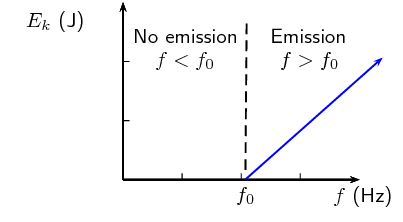It can be seen from the graph that $$E_{k}$$ is plotted on the $$y-$$axis and $$f$$ is plotted on the $$x-$$axis. Using the straight line equation, $$y=mx+c$$, we can identify

\begin{align*} {E}_{k\max}& = hf-W_{0} \\ \underbrace{{E}_{k\max}}_{y}& = h\underbrace{f}_x-hf_{0} \end{align*} This allows us to conclude that the slope of the graph $$m$$ is Planck's constant $$h$$. Also, the $$x$$ intercept is the cut-off frequency $$f_{0}$$.

## Worked example 1: The photoelectric effect using silver

Ultraviolet radiation with a wavelength of $$\text{250}$$ $$\text{nm}$$ is incident on a silver foil (work function $$W_0$$ = $$\text{6,9} \times \text{10}^{-\text{19}}$$ $$\text{J}$$). What is the maximum kinetic energy of the emitted electrons?

### Determine what is required and how to approach the problem

We need to determine the maximum kinetic energy of an electron ejected from a silver foil by ultraviolet radiation.

The photoelectric effect tells us that:

\begin{align*} {E}_{k\max}& = {E}_{\mathrm{photon}}-W_0 \\ {E}_{k\max}& = h\frac{c}{\lambda }-W_0 \end{align*}

We also have:

• Work function of silver: $$W_{0~\text{silver}} =\text{6,9} \times \text{10}^{-\text{19}}\text{ J}$$
• UV radiation wavelength = $$\text{250}$$ $$\text{nm}$$ = $$\text{250} \times \text{10}^{-\text{9}}$$ $$\text{m}$$ = $$\text{2,50} \times \text{10}^{-\text{7}}$$ $$\text{m}$$
• Planck's constant: $$h=\text{6,63} \times \text{10}^{-\text{34}}\text{ m^{2}·kg·s^{-1}}$$
• Speed of light: $$c=\text{3} \times \text{10}^{\text{8}}\text{ m·s^{-1}}$$

### Solve the problem

\begin{align*} {E}_{k}& = \frac{hc}{\lambda }-W_{0~\text{silver}} \\ & = \left[ \text{6,63} \times \text{10}^{-\text{34}} \times \frac{\text{3} \times \text{10}^{\text{8}}}{\text{2,5} \times \text{10}^{-\text{7}}}\right]-\text{6,9} \times \text{10}^{-\text{19}}\\ & = \text{1,06} \times \text{10}^{-\text{19}}\text{ J} \end{align*}

The maximum kinetic energy of the emitted electron will be $$\text{1,06} \times \text{10}^{-\text{19}}$$ $$\text{J}$$.

## Worked example 2: The photoelectric effect using gold

If we were to shine the same ultraviolet radiation ($$f=\text{1,2} \times \text{10}^{\text{15}}\text{ Hz}$$) on a gold foil (work function = $$\text{8,2} \times \text{10}^{-\text{19}}$$ $$\text{J}$$ ) would any electrons be emitted from the surface of the gold foil?

### Calculate the energy of the incident photons

For the electrons to be emitted from the surface, the energy of each photon needs to be greater than the work function of the material.

\begin{align*} {E}_{\mathrm{photon}}& = hf\\ & = \text{6,63} \times \text{10}^{-\text{34}}\times \text{1,2} \times \text{10}^{\text{15}}\\ & = \text{7,96} \times \text{10}^{-\text{19}}\text{ J} \end{align*}

Therefore each photon of ultraviolet light has an energy of $$\text{7,96} \times \text{10}^{-\text{19}}$$ $$\text{J}$$.

### Write down the work function for gold.

\begin{align*} W_{0~\mathrm{gold}}& = \text{8,92} \times \text{10}^{-\text{19}}\text{ J} \end{align*}

### Is the energy of the photons greater or smaller than the work function?

\begin{align*} \text{7,96} \times \text{10}^{-\text{19}}\text{ J}& < \text{8,92} \times \text{10}^{-\text{19}}\text{ J}\\ {E}_{\mathrm{photons}}& < W_{0~\mathrm{gold}} \end{align*}

Since the energy of each photon is less than the work function of gold, the photons do not have enough energy to knock electrons out of the gold. No electrons would be emitted from the gold foil.

## Worked example 3: [NSC 2011 Paper 1]

A metal surface is illuminated with ultraviolet light of wavelength $$\text{330}$$ $$\text{nm}$$. Electrons are emitted from the metal surface.

The minimum amount of energy required to emit an electron from the surface of this metal is $$\text{3,5} \times \text{10}^{-\text{19}}$$ $$\text{J}$$.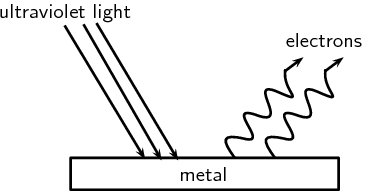1. Name the phenomenon illustrated above.

(1 mark)

2. Give ONE word or term for the underlined sentence in the above paragraph.

(1 mark)

3. Calculate the frequency of the ultraviolet light.

(4 marks)

4. Calculate the kinetic energy of a photoelectron emitted from the surface of the metal when the ultraviolet light shines on it.

(4 marks)

5. The intensity of the ultraviolet light illuminating the metal is now increased. What effect will this change have on the following:

1. Kinetic energy of the emitted photoelectrons (Write down only INCREASES, DECREASES or REMAINS THE SAME.)

(1 mark)

2. Number of photoelectrons emitted per second (Write down only INCREASES, DECREASES or REMAINS THE SAME.)

(1 mark)

6. Overexposure to sunlight causes damage to skin cells.

1. Which type of radiation in sunlight is said to be primarily responsible for this damage?

(1 mark)

2. Name the property of this radiation responsible for the damage.

(1 mark)

[TOTAL: 14 marks]

Question 1

Photo-electric effect

(1 mark)

Question 2

Work function

(1 mark)

Question 3

\begin{align*} c & = f \lambda \\ \text{3} \times \text{10}^{\text{8}} & = f(\text{330} \times \text{10}^{-\text{9}})\\ \therefore f & = \text{9,09} \times \text{10}^{\text{14}}\text{ Hz} \end{align*}

OR

\begin{align*} E & = \frac{hc}{\lambda} \\ & = \frac{(\text{6,63} \times \text{10}^{-\text{34}})(\text{3} \times \text{10}^{\text{8}})}{(\text{330} \times \text{10}^{-\text{9}})} \\ & = \text{6,03} \times \text{10}^{-\text{19}}\text{ J} \end{align*}\begin{align*} E & = hf \\ \text{6,03} \times \text{10}^{-\text{19}}\text{ J} & = (\text{6,63} \times \text{10}^{-\text{34}})f\\ \therefore f & = \text{9,09} \times \text{10}^{\text{14}}\text{ Hz} \end{align*}

(4 marks)

Question 4

Option 1:

\begin{align*} E & = W_{o} + K \\ \frac{hc}{\lambda} & = W_{o} + K \\ \therefore \frac{(\text{6,63}\times10^{-34})(3\times10^8)}{330\times10^{-9}} & = \text{3,5}\times10^{-19} + K\\ \therefore K & = \text{2,53}\times10^{-19}\text{ J} \end{align*}

Option 2:

\begin{align*} E & = W_{o} + K \\ hf & = W_{o} + K \\ \therefore (\text{6,63}\times10^{-34})(\text{9,09}\times10^{14} & = \text{3,5}\times10^{-19} + K\\ \therefore K & = \text{2,53}\times10^{-19}\text{ J} \end{align*}

(4 marks)

Question 5.1

Remains the same.

(1 mark)

Question 5.2

Increases

(1 mark)

Question 6.1

(1 mark)

Question 6.2

High energy or high frequency

(1 mark)

[TOTAL: 12 marks]

temp text

### Solar cells (ESCQQ)

The generation of electricity using solar cells isn't due to the photoelectric effect but is similar in nature. Photons in sunlight hit the solar panel and are absorbed by semiconducting materials, such as silicon.

Electrons (negatively charged) are knocked loose from their atoms, allowing them to flow through the material to produce electricity. This is called the photovoltaic effect. The photovoltaic effect was first observed by French physicist Antoine E. Becquerel in 1839.

Due to the special composition of solar cells, the electrons are only allowed to move in a single direction. An array of solar cells converts solar energy into a usable amount of direct current (DC) electricity.

## The photoelectric effect

Textbook Exercise 12.1

Describe the photoelectric effect.

The photoelectric effect its the process whereby an electron is emitted by a metal when light shines on it, on the condition that the energy of the photons (light energy packets) are greater than or equal to the work function of the metal.

List two reasons why the observation of the photoelectric effect was significant.

Two reasons why the observation of the photoelectric effect was significant are (1) that it provides evidence for the particle nature of light and (2) that it opened up a new branch for technological advancement e.g. photocathodes (like in old TVs) and night vision devices.

Refer to Table 12.1: If I shine ultraviolet light with a wavelength of $$\text{288}$$ $$\text{nm}$$ onto some aluminium foil, what would the kinetic energy of the emitted electrons be?

\begin{align*} E_k & = E_{photon} - W_0 \\ & = h\frac{c}{\lambda} - W_0 \\ & = (\text{6,63} \times \text{10}^{-\text{34}}\text{ m$^{2}$kg·s$^{-1}$})\frac{\text{3} \times \text{10}^{\text{8}}\text{ m·s$^{-1}$}}{\text{288} \times \text{10}^{-\text{9}}\text{ m}} - \text{6,9} \times \text{10}^{-\text{19}}\text{ J} \\ & = \text{6,25} \times \text{10}^{-\text{22}}\text{ J} \end{align*}

$$\text{6,25} \times \text{10}^{-\text{22}}$$ $$\text{J}$$

I shine a light of an unknown wavelength onto some silver foil. The light has only enough energy to eject electrons from the silver foil but not enough to give them kinetic energy. (Refer to Table 12.1 when answering the questions below:)

1. If I shine the same light onto some copper foil, would electrons be ejected?

2. If I shine the same light onto some silicon, would electrons be ejected?

3. If I increase the intensity of the light shining on the silver foil, what happens?

4. If I increase the frequency of the light shining on the silver foil, what happens?

1. The kinetic energy of the ejected photon is given by \begin{align*} E_k & = E_{photon} - W_0 \end{align*} and if for silver foil $$E_k = 0$$ then $$E_{photon} = W_{0~\text{silver}}$$. Therefore, if the same light falls on copper foil we require $$E_{photon} > W_{0~\text{copper}}$$ for electrons to be ejected.

The work function for silver is $$W_{0~\text{silver}} = \text{6,9} \times \text{10}^{-\text{19}}\text{ J}$$ and the work function for copper is $$W_{0~\text{copper}} = \text{7,5} \times \text{10}^{-\text{19}}\text{ J}$$. Now \begin{align*} W_{0~\text{silver}} & < W_{0~\text{copper}} \\ ∴ E_{photon} &< W_{0~\text{copper}} \end{align*} and thus no electrons will be ejected.

2. Similarly to the previous question we know the kinetic energy of the ejected photon is given by \begin{align*} E_k & = E_{photon} - W_0 \end{align*} and if for silver foil $$E_k = 0$$ then $$E_{photon} = W_{0~\text{silver}}$$. Therefore, if the same light falls on copper foil we require $$E_{photon} > W_{0~\text{silicon}}$$ for electrons to be ejected.

The work function for silver is $$W_{0~\text{silver}} = \text{6,9} \times \text{10}^{-\text{19}}\text{ J}$$ and the work function for silicon is $$W_{0~\text{silicon}} = \text{1,8} \times \text{10}^{-\text{19}}\text{ J}$$. Now \begin{align*} W_{0~\text{silver}} & > W_{0~\text{silicon}} \\ ∴ E_{photon} & > W_{0~\text{silicon}} \end{align*} and thus electrons will be ejected.

3. More electrons will be ejected from the silver.
4. Increasing the frequency of the light would increase $$E_{photon}$$ and therefore $$E_{photon} > W_{0~\text{silver}}$$ which means that the electron will be ejected with a kinetic energy of $$E_k = E_{photon} - W_{0~\text{silver}} > 0$$.

The following results were obtained from a photoelectric effect experiment.

 $$f~(\times 10^{15}~\text{Hz})$$ $$E_{k}~(\times 10^{-19}~\text{J})$$ 0.60 0.24 0.80 1.59 1.00 2.89 1.20 4.20 1.40 5.55 1.60 6.89
1. Plot a graph of $$E_{k}$$ on the $$y$$ axis and $$f$$ on the x axis
2. Calculate the gradient of the graph.
3. The metal used in the experiment is Sodium which has a work function of $$3,7 \times 10^{-19}$$. Calculate the cut-off frequency for sodium.
4. Determine the $$x$$ intercept. Compare your $$x$$ intercept value with the cut-off frequency you calculated.
5. If the sodium metal was replaced with another metal with double the work function, sketch on the same graph for sodium, the result you would expect to obtain.
1. gradient = $$6.6 \times 10^{-34}$$
2. \begin{align*} W_{0}&=h~f_{0} \\ 3.7 \times 10^{-19} &= 6.6 \times 10^{-34} f_{0} \\ f_0 &= 0.56 \times 10^{15} ~\text{Hz} \end{align*}
3. Check that your $$x$$ intercept is close to $$\text{0,56} \times \text{10}^{\text{15}}$$ which is the cut-off frequency